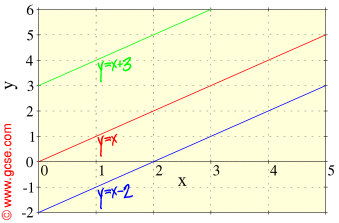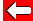# Higher or Lower GraphsAnother way of drawing different x-y graphs is to make the line higher or lower than y=x:The green line y=x+3 above is higher up than the line y=x because each value of y on the line is 3 more than that of x.

However, the blue line y=x-2 above is lower down than the line y=x because each value of y on the line is 2 less that of x.

The number after x in the formula tells us how high the line will be. Positive values raise the line, negative values lower it.

N.B. Notice that all three lines above have the same gradient.Go back a pageMaths MenuGo to next page